Zhang, J.-L., Wang, G.-W., Shao, J.-G., and Zuo, H.-B. (2014). "A modified random pore model for the kinetics of char gasification," BioRes. 9(2), 3497-3507.

#### Abstract

Based on traditional kinetic models of the gasification process of char, a new modified random pore model (MRP) was proposed. This model can be reduced to a traditional volume model (VM), an unreacted shrinking core model (URCM), a hybrid model (HM), and a random pore model (RPM) by varying the model parameters. Furthermore, not only is the relationship between the reaction rate and conversion rate well described by MRP, as it is in other models, but the position of the maximum reaction rate is also described, which is out of the application range of other traditional models. MRP was validated by gasification of different kinds of chars under different experimental conditions, such as in a carbon dioxide atmosphere, in the presence of water vapor, or with the addition of catalyst. The char gasification process under various conditions could be simulated by MRP with better fitting results than the traditional RPM.

#### Full Article

A Modified Random Pore Model for the Kinetics of Char Gasification

Jian-Liang Zhang,a Guang-Wei Wang,a,* Jiu-Gang Shao,a and Hai-Bin Zuo b

Based on traditional kinetic models of the gasification process of char, a new modified random pore model (MRP) was proposed. This model can be reduced to a traditional volume model (VM), an unreacted shrinking core model (URCM), a hybrid model (HM), and a random pore model (RPM) by varying the model parameters. Furthermore, not only is the relationship between the reaction rate and conversion rate well described by MRP, as it is in other models, but the position of the maximum reaction rate is also described, which is out of the application range of other traditional models. MRP was validated by gasification of different kinds of chars under different experimental conditions, such as in a carbon dioxide atmosphere, in the presence of water vapor, or with the addition of catalyst. The char gasification process under various conditions could be simulated by MRP with better fitting results than the traditional RPM.

Keywords: Modified random pore model; Char; Gasification

Contact information: a: School of Metallurgical and Ecological Engineering, University of Science and Technology Beijing, Beijing 100083, China; b: State Key Laboratory of Advanced Metallurgy, University of Science and Technology Beijing, Beijing 100083, China; *Corresponding author: wgw676@163.com

INTRODUCTION

Gasification is a promising conversion technology that can provide chemicals and energy from various fossil and renewable sources. Gasification can be divided into two main stages: pyrolysis and the subsequent gasification of the remaining char, the latter stage known to be the controlling step for overall conversion process because its reaction rate is much slower than the former step. Therefore, successful reactivity and kinetic modeling studies have a close connection with the improvement of gasifier design and process efficiency.

To date, various models have been proposed by different researchers for the gasification process of char, such as the volume model (VM) (Kasaoka et al. 1984; Shang and Eduardo 1984), the unreacted shrinking core model (URCM) (Wen 1968), the hybrid model (HM) (Shuai et al. 2013; Xiang et al. 2002), and the random pore model (RPM) (Bhatia and Perlmutter 1980; Bhatia and Perlmutter 1981). Among these, the most prominent one is the RPM, which considers the effects of pore growth and coalescence during reaction and can describe the maximum reaction rate at low conversion levels. RPM has been used to study the gasification process of coal char, pyrolysis char of tires, and biomass char (Aranda et al. 2007; Fermoso et al. 2008; Kajita et al. 2010; Marillo et al. 2004; Micco et al. 2012; Ochoa et al. 2001; Yang et al. 2005). However, there are obvious deviations between experimental data and the calculated results by the traditional RPM when used for the gasification reactions of biomass char and coal char, especially for cases in which the peak reaction rate is reached at the high conversion rate stage. Some modifications were made to the traditional RPM to improve its suitability for various applications (Bhatia and Vartak 1996; Gupta and Bhatia 2000; Liu et al. 2003; Strais et al. 2002).

The primary objective of this paper was to find a simple model that could be applied to biomass char and coal char gasification under a carbon dioxide atmosphere or in the presence of water vapor. For this purpose, the following investigations were performed. First, based on VM, HM, and RPM analysis, a new modified random pore model (MRP) was proposed. Second, a non-linear fitting method was used to calculate kinetic parameters. Finally, experimental data obtained from the literature was used to prove the validity of the new model.

EXPERIMENTAL

Methods

Kinetics model

Because of the heterogeneous structure of char, the gasification process is complicated. Different chars and different reaction conditions often call for different kinetic models. A general kinetic expression for the overall reaction rate is given as follows (Zhang et al. 2010),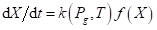(1)

where k is the apparent gasification reaction rate, which includes the effects of reaction temperature (T) and partial pressure in the gas phase (Pg), and (X) describes the changes in physical or chemical properties of the samples as the gasification proceeds. If the partial pressure in the gas phase remains constant during the gasification process, the temperature dependence of apparent reaction rate constant can be expressed by the Arrhenius equation as follows,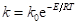(2)

where ko is the pre-exponential factor, also called the frequency factor, E stands for activation energy, kJ/mol, and R is the universal gas constant.

VM, URCM, HM, and RPM are widely used models for the gasification process of char. These models give different formulations of the term (X). For the VM model, the gasification reaction is assumed to occur inside the particle, and the size of the particle does not change during the reaction process, although the density changes uniformly. When the reaction is of the first order, the formula for the reaction rate is,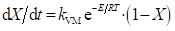(3)

where kVM denotes the reaction rate constant.

In the URCM model, the reaction occurs on the surface of the particle, and there is an obvious interface between product and unreacted core. When the reaction is under chemical reaction control, the formula for the reaction rate is,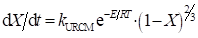(4)

where kURCM denotes the reaction rate constant.

Because of the complexity of the gasification of char, neither VM nor URCM can describe the reaction process satisfactorily, so HM has been proposed. Empirical factors are considered by HM, and the common form is,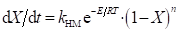(5)

where kHM denotes the reaction rate constant and n is an empirical constant.

RPM assumes the pore structure of char particles consists of many cylindrical holes having different diameters, and the reaction occurs from the surfaces of the holes. During the reaction process, pores expand and overlap with each other. The formula for RPM is,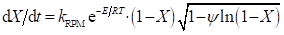(6)

where kRPM denotes the reaction rate constant and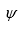is a structural constant (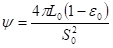), where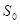,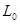, and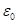are the pore surface area, pore length, and solid porosity, respectively).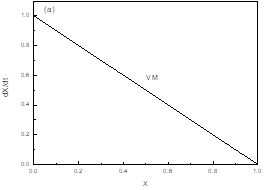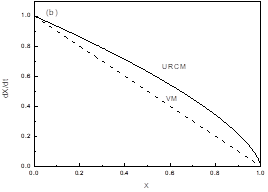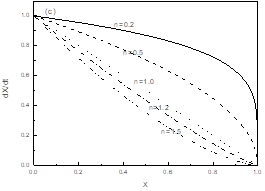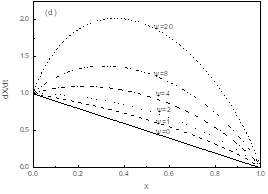Fig. 1. Dimensionless reaction rate-conversion curves described by the models (a) VM, (b) URCM, (c) HM, and (d) RPM

The relationships between the reaction rates and conversion rates of different models are shown in Fig. 1. The reaction rate of VM linearly decreases with an increase in conversion rate (Fig. 1a), while the reaction rate of URCM also decreases，but the decreasing tendency increases with increasing of the conversion rate (Fig. 1b). The result of HM is shown in Fig. 1c, and the reaction rate also decreases with an increasing conversion rate. Furthermore, according to different values of n, two trends can be distinguished; in the case of < 1, the decreasing tendency of the reaction rate gradually increases and the maximum value is reached at the last stage of conversion, while in the case of > 1, the decreasing tendency gradually decreases and the maximum value is reached at the initial stage of conversion. By comparing Eqs. 3, 4, and 5, when n in the HM model is assigned the value of 2/3, HM and URCM hold the same form; when n in the HM model is assigned the value of 1, HM and VM hold the same form. Therefore, the semiempirical VM model has more application relevancy according to the adjustable parameter n. The relationship between the reaction rate and conversion rate for RPM is shown in Fig. 1d. The reaction rate first increases and then decreases, and with increasing value of ψ, the maximum reaction rate is reached at a higher conversion rate range, with calculation formula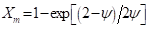. The relationship between ψ and Xis shown in Fig. 2. With an increase in the structure parameter ψ, the maximum reaction rate is reached at a higher conversion rate, but the increasing trend of Xis diminishing, with an extreme value for Xof 0.393. However, when the value of Xin practical reaction processes is over 0.393, the traditional RPM is no longer effective.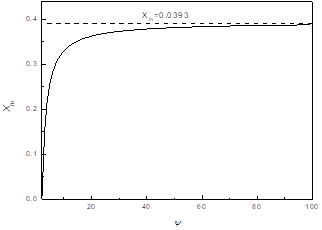Fig. 2. The change in Xm with ψ for RPM

In VM, URCM, and HM, the reaction rate decreases as the conversion rate increases and therefore cannot be used when that reaction rate first increases and then decreases. The RPM can be applied to this case, when X< 0.393, but it is invalid when X> 0.393. If the item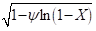in the RPM formula is removed, it has the same form as VM, so RPM can be considered as a modification of VM. To extend the application range of RPM, a further modification must be performed on RPM: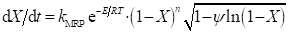(7)

In Eq. 7, an exponent factor is added to modify RPM in the same manner that HM modifies VM. In the Eq. (7), parameter n is an empirical factor, which is influenced by shape structure of solid reactant, porosity, different catalysts, and gaseous reactants.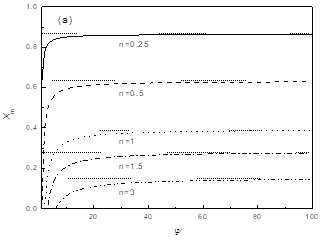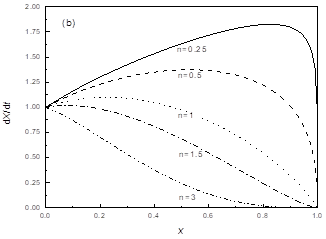Fig. 3. (a) The relationship between Xand ψ for MRP with differentn values, and (b) the changes in dX/dwith X for MRP (ψ=4)

Using Eq. (7), the relationship between Xm and ψ with different nvalues is shown in Fig 3a. With a decrease in n from 3 to 0.25, the range of Xincreases from 0 to 0.153 to 0 to 0.865. In Fig 3b, the changes in dX/dwith are shown in the case of ψ = 4, with varying from 3 to 0.25. With a decrease in n, the maximum reaction rate point moves into a higher conversion rate. MRP is founded on the basis of RPM and HM, so the characteristics of MRP can be summarized as follows: for ψ = 0, MRP is the same as HM; for = 1, MRP is the same as the traditional RPM; for ψ = 0, = 1, MRP is the same as VM; for ψ = 0, = 2/3, MRP is the same as URCM. Therefore, it can be concluded that VM, URCM, HM, and RPM can all be described by MRP at different values of ψ and n. Additionally, cases where the maximum reaction rate occurs at a high conversion rate range that is out of the application range of other models can also be described by MRP.

Equations 6 and 7 can be utilized to determine kE, and ψ from experimental data sets by employing nonlinear least-squares fitting methods. As pointed out by Miura and Silveston (1989), the determinations of the kinetic parameters from a single temperature-programmed reaction run may lead to unreliable rate parameters, and the validation of the model thus cannot be well assessed if using only one temperature-programmed reaction run; at least three temperature-programmed reaction runs at different heating rates are required to estimate reliable parameters and accurate activation energies (Miura and Silveston 1989). This should be considered in isothermal experiments.

In this study, the kinetic parameters were determined from at least three kinetic curves obtained at different temperatures. The nonlinear least-squares method was employed to fit the experimental data of dX/dt vs. the conversion rate X, which was carried out on a program compiled by author with C++ programming language, and the kinetic parameters (kE, n, and ψ) in RPM and MRP were determined by minimizing the sum of squares of the relative error (SSRE), defined as,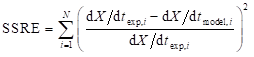(8)

where dX/dtexp,i is the experimental data corresponding to the conversion rate Xi, dX/dtmodel,i is the value calculated at the conversion rate Xi, and N is the number of data points.

RESULTS

In this work MRP was used to study the gasification of char under different conditions, and the results were compared with traditional RPM. Experimental data were obtained from the literature (Chodankar et al. 2010; Fan 2013; Lahijani and Zainal 2013a, b; Wang 2011; Woodruff and Weimer 2013).

Application of MRP in Gasification of Char under CO2 Atmosphere

Coal char gasification under different concentrations of COwas studied by Chodankar et al. (2010). In Fig. 4a, gasification kinetic curves under an atmosphere of 20% CO2 and 80% Nfor char A (with particle sizes in the range of 180 to 212 μm) are shown. The reaction rate first increased and then decreased with increasing conversion rate. The maximum reaction rate occurred at a conversion rate of around X = 0.2. RPM and MRP were used to simulate the reaction process shown in Fig. 4a. All of the optimized parameters are included in Table 1. Both RPM and MRP show good estimation results, with correlation coefficients (R2) of 0.9920 and 0.9926, respectively. Therefore, gasification of char A can be described by both RPM and MRP.

Gasification of SGY-RP900 coal char under CO2 conditions was studied by Fan (2013), as shown in Fig. 4b. The reaction rate first quickly increased with increasing conversion rate; the reaction rate then changed slowly within the conversion rate range of 20 to 80%, but the reaction rate decreased quickly when the conversion rate was higher than 80%. From the fitting results of RPM and MRP shown in Fig. 4b, MRP gave much better results.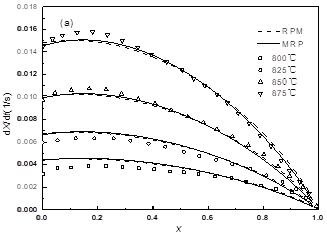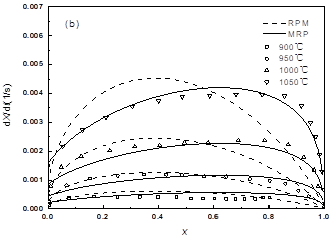Fig. 4. A comparison of coal char gasification using experimental data and theoretical predictions by RPM and MRP: (a) char A and (b) SGY-RP900 char at different temperatures

Table 1. Kinetic Parameters for the Gasification of Coal Char using RPM and MRP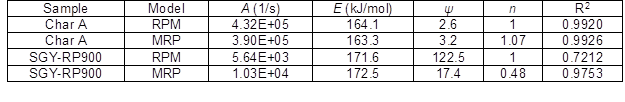Gasification tests of coal char and pine wood char were performed in a thermogravimetric analyzer (HCT-3, Henven Scientific Instrument Factory, Beijing) with the gasification agent CO2 (purity>99.999%) at the temperatures of 900 oC, 950 oC, and 1000 oC. Results of tests are shown in Fig. 5. For all temperatures, the reaction rates of coal char and pine wood char first increased and then decreased. Peak rates of gasification for coal char and pine wood char appeared in the ranges of X<0.393 and X>0.393, respectively. RPM and MRP models are used to fit with experiment data, as shown in Fig. 5. It could be concluded that both models could be used to describe gasification of coal char, but only MRP could be used to describe gasification of pine wood char. The kinetic parameters of the two kinds of char calculated by the two models are shown in Table 2. Activation energies of coal char and pine wood char obtained by MRP kinetic model were 194.3kJ/mol and 123.3kJ/mol, respectively, which are consistent with values reported in previous works provided by Miccoet al. (2012) and Yuan et al. (2011).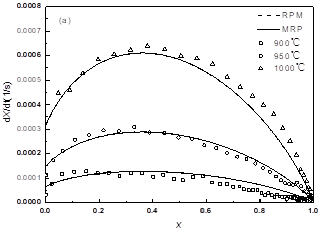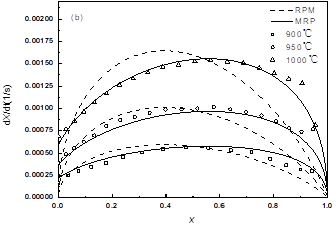Fig. 5. A comparison of coal char and pine wood char gasification using experimental data and theoretical predictions by RPM and MRP: (a) Coal char and (b) pine wood char at different temperatures

Table 2. Kinetic Parameters for the Gasification of Coal Char and Pine Wood Char by using RPM and MRP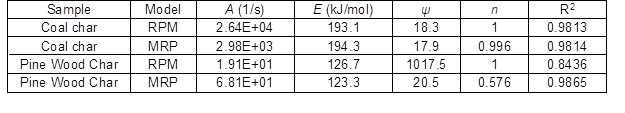Application of MRP in Gasification of Char under Water Vapor Atmosphere

Gasification of switchgrass char under water vapor and hydrogen conditions was studied by Woodruff and Weimer (2013). The relationship between gasification rate and conversion rate at 1000, 1075, and 1150 °C is shown in Fig. 6a. Gasification of petroleum char under water vapor conditions was studied by Wang (2011), as shown in Fig. 6b. For both kinds of char, the reaction rates first increased and then decreased with increasing conversion rate; for switchgrass char, the conversion rate at which the maximum reaction rate was reached was lower than that of petroleum char. RPM and MRP were used to fit the experimental data of the two kinds of char, and the corresponding results are also shown in Fig. 6. The fit of MRP is obviously better than that of RPM. The kinetic parameters of the two models are shown in Table 3. For switchgrass char, the MRP parameters are = 1.30, ψ = 33.4, and = 133.7 kJ/mol, with R= 0.9917. For petroleum char, the MRP parameters were = 0.81, ψ = 48.0, and E = 88.5 kJ/mol, with R= 0.9912.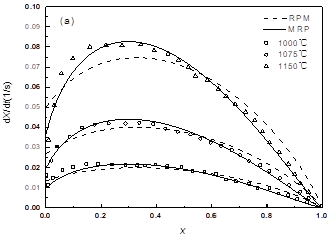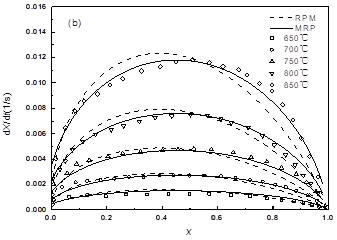Fig. 6. A comparison of gasification using experimental data and theoretical predictions by RPM and MRP: (a) switchgrass char and (b) petroleum char at different temperatures

Table 3. Kinetic Parameters for the Gasification of Switchgrass Char and Petroleum Char by RPM and MRP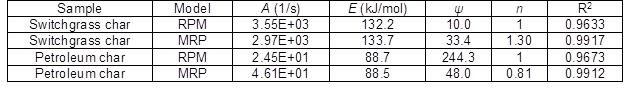Application of MRP in Catalytic Gasification of Char

Lahijani and Zainal (2013a) investigated the co-gasification of tires and biomass for enhancement of tire-char reactivity in the CO2gasification process. Gasification kinetic curves of the mixtures of 50% tire char and 50% almond shell (Tire-AS) are shown in Fig. 7a. The gasification rate first increased and then decreased with increasing conversion rate. The maximum gasification rate occurred at a high conversion rate, while this maximum rate must occur in the conversion rate range of 0 to 0.393 for traditional RPM. Gasification of pistachio nut shell (PNS) char under COconditions with different catalysts was studied by Lahijani and Zainal (2013b). Data from the gasification of PNS char with 5% Na addition at different temperatures are shown in Fig. 7b, in which the maximum reaction rate occurred at a conversion rate of around 0.2. RPM and MRP were used to fit the experimental data for both kinds of char. For Tire-AS char, the MRP fitting result was better than that of RPM, and for PNC char, both the two models had a good fitting result. Kinetic calculation results are shown in Table 4.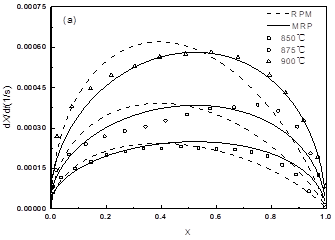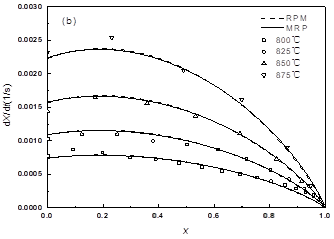Fig. 7. A comparison of gasification using experimental data and theoretical predictions by RPM and MRP: (a) Tire-AS blend char and (b) PNS char-5% Na at different temperatures

Table 4. Kinetic Parameters for the Gasification of Tire-AS Char and PNC Char by RPM and MRP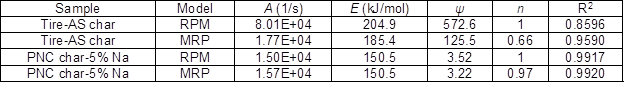DISCUSSION

Gasification of char is a complex process, and many kinetic models have been proposed to describe it. Among them, RPM is the most widely used model. However, RPM cannot be used when the maximum gasification rate occurs at a high conversion rate. The new proposed MRP can overcome this shortcoming. MRP can be reduced to VM, URCM, HM, and RPM by adjusting the model parameters. Compared with the traditional method for calculating activation energy, the nonlinear fitting method used in the present study is a relatively simple one-step regression method. Take char A in Fig. 4a, for example: in the traditional method, four times of data regressions at different temperatures should be first carried out to calculate k, and then lnk is plotted as a function of 1/T to calculate the activation energy E, so a total of five regressions should be applied. For the method used in this study, only one regression, following Eq. 8, is needed to calculate kinetic parameters. MRP may be used not only in the gasification of char, but should also be applicable in other research fields such as the combustion of char and the reduction of porous media, as these processes have a similar reaction mechanism.

CONCLUSIONS

1. The RPM, which is widely used to model the kinetics of char gasification, cannot be used when the maximum gasification rate occurs at a high conversion rate. This study proposes a new MRP model that can overcome this shortcoming and can be reduced to different kinetic models, e.g., VM, URCM, HM, and RPM, by changing the model parameters.
2. According to the fitting results for MRP and RPM, MRP has a much higher accuracy in describing the gasification of different chars under different experimental conditions.

ACKNOWLEDGMENTS

The present work was supported by the National Science Foundation of China and Baosteel under Grant 51134008 and by the National Key Technology R&D Program in the 12th Five Year Plan of China (No. 2011BAC01B02).

REFERENCES CITED

Aranda, A., Murillo, R., García, T., Callén, M. S., and Mastral, A. M. (2007). “Steam activation of tyre pyrolytic carbon black: Kinetic study in a thermoblance,” Chemical Engineering Journal 126(2-3), 79-85.

Bhatia, S. K., and Perlmutter, D. D. (1980). “A random pore model for fluid-solid reactions: І. Isothermal kinetic control,” AIChE Journal 26(3), 379-385.

Bhatia, S. K., and Perlmutter, D. D. (1981). “A random pore model for fluid-solid reactions: ІІ. Diffusion and transport effects,” AIChE Journal 27(2), 247-254.

Bhatia, S. K., and Vartak, B. J. (1996). “Reaction of microporous solid: The discrete random pore model,” Carbon 34(11), 1383-1391.

Chodankar, C. R., Feng, B., Ran, J., and Klimenko, A. Y. (2010). “Kinetic study of the gasification of an Australian bituminous coal in carbon dioxide,” Asia-Pacific Journal of Chemical Engineering 5(3), 413-419.

Fan, D. M. (2013). Characteristics Study on Pyrolysis and Gasification of Low Rank Coals, Ph.D. thesis, University of Chinese Academy of Sciences, Beijing, China.

Fermoso, J., Arias, B., Pevida, C., Plaza, M. G., Rubiera, F., and Pis, J. J. (2008). “Kinetic models comparison for steam gasification of different nature fuel chars,” Journal of Thermal Analysis and Calorimetry 91(3), 779-786.

Gupta, J. S., and Bhatia, S. K. (2000). “A modified discrete random pore model allowing for different initial surface reactivity,” Carbon38(1), 47-58.

Kajita, M., Kimura, T., Norinaga, K., Li, C., and Hayashi, J. (2010). “Catalytic and noncatalytic mechanisms in steam gasification of char form the pyrolysis of biomass,” Energy Fuels 24(1), 108-116.

Kasaoka, S., Sakata, Y., and Tong, C. (1984). “Kinetic evaluation of reactivity of various coal chars for gasification with carbon dioxide in comparison with stream,” International Chemical Engineering 25(1), 160-175.

Lahijani, P., Zainal, Z. A., Mohamed, A. R., and Mohammadi, M. (2013a). “Co-gasification of tire and biomass for enhancement of tire-char reactivity in CO2 gasification process,” Bioresource Technology138,124-130.

Lahijani, P., Zainal, Z. A., Mohamed, A. R., and Mohammadi, M. (2013b). “CO2 gasification reactivity of biomass char: Catalytic influence of alkali, alkaline earth and transition metal salts,” Bioresource Technology 144, 288-295.

Liu, H., Lao, C. H., Kaneko, M., Kato, S., and Kojima, T. (2003). “Unification of gasification kinetics of char in CO2 at elevated temperatures with a modified random pore model,” Energy Fuels17(4), 961-970.

Marillo, R., Navarro, M. V., López, J. M., Garćıa, T., Callén, M. S., Aylón, E., Mastral, A. M. (2004). “Activation of pyrolytic tire char with CO2: Kinetic study,” Journal of Analytical and Applied Pyrolysis 71(2), 945-957.

Micco, G. D., Nasjleti, A., and Bohé, A. E. (2012). “Kinetic of the gasification of a Rio Turbio coal under different pyrolysis temperatures,” Fuel 95(1), 537-543.

Miura, K., and Silveston, P. L. (1989). “Analysis of gas-solid reaction by use of a temperature-programmed reaction technique,” Energy Fuels 3(2), 243-249.

Ochoa, J., Casanello, M. C., Bonelli, P. R., and Cukerman, A. L. (2001). “CO2 gasification of Argentinean coal chars: A kinetic characterization,” Fuel Process Technology 74(3), 161-76.

Shang, J. Y., and Eduardo, E. W. (1984). “Kinetic and FTIR studies of the sodium-catalyzed steam gasification of coal char,” Fuel 63(11), 1604-1609.

Shuai, C., Bin, Y. Y., Hu, S., Xiang, J., Sun, L. S., Su, S., Xu, K., and Xu, C. F. (2013). “Kinetic models of coal char steam gasification and sensitivity analysis of the parameters,” Journal of Fuel Chemistry and Technology 41(5), 558-564.

Strais, R. P. M. J., Scala, C., Stucki, S., and Prins, R. (2002). “Gasification reactivity of char coal with CO2. Part I: Conversion and structural phenomena,” Chemical Engineering Science 57(17), 3581-3592.

Wang, J. J. (2011). Study on Catalytic Gasification Characteristics of Petroleum Coke, M.S. thesis, East China University of Science and Technology, Liaoning, China.

Wen, C. Y. (1968). “Non-catalytic heterogeneous solid-fluid reaction models,” Industrial and Engineering Chemistry 60(9), 34-54.

Woodruff, R. B., and Weimer, A. W. (2013). “A novel technique for measuring the kinetics of high-temperature gasification of biomass char with steam,” Fuel 103, 749-757.

Xiang, Y., Wang, Y., Zhang, J., Huang, J., and Zhao, J. (2002). “A study on kinetic models of char gasification,” Journal of Fuel Chemistry and Technology 30(1), 21-26.

Yang, F., Fan, X. L., Zhou, Z. J., Liu, H. F., Gong, X., and Yu, Z. H. (2005). “Kinetics of coal char gasification with CO2 random pore model,” Journal of Fuel Chemistry and Technology 33(6), 671-676.

Yuan, S., Chen, X. L., Li, J., and Wang, F. C. (2011). “CO2gasification kinetics of biomass char derived from high-temperature rapid pyrolysis,” Energy Fuels 25(5), 2314-2321.

Zhang, Y., Hara, S., Kajitani, S., and Ashizawa, M. (2010). “Modeling of catalytic gasification kinetics of coal char and carbon,”Fuel 89(1), 152-157.

Article submitted: December 29, 2013; Peer review completed: March 27, 2014; Revised version received and accepted: April 23, 2014; Published: April 29, 2014.